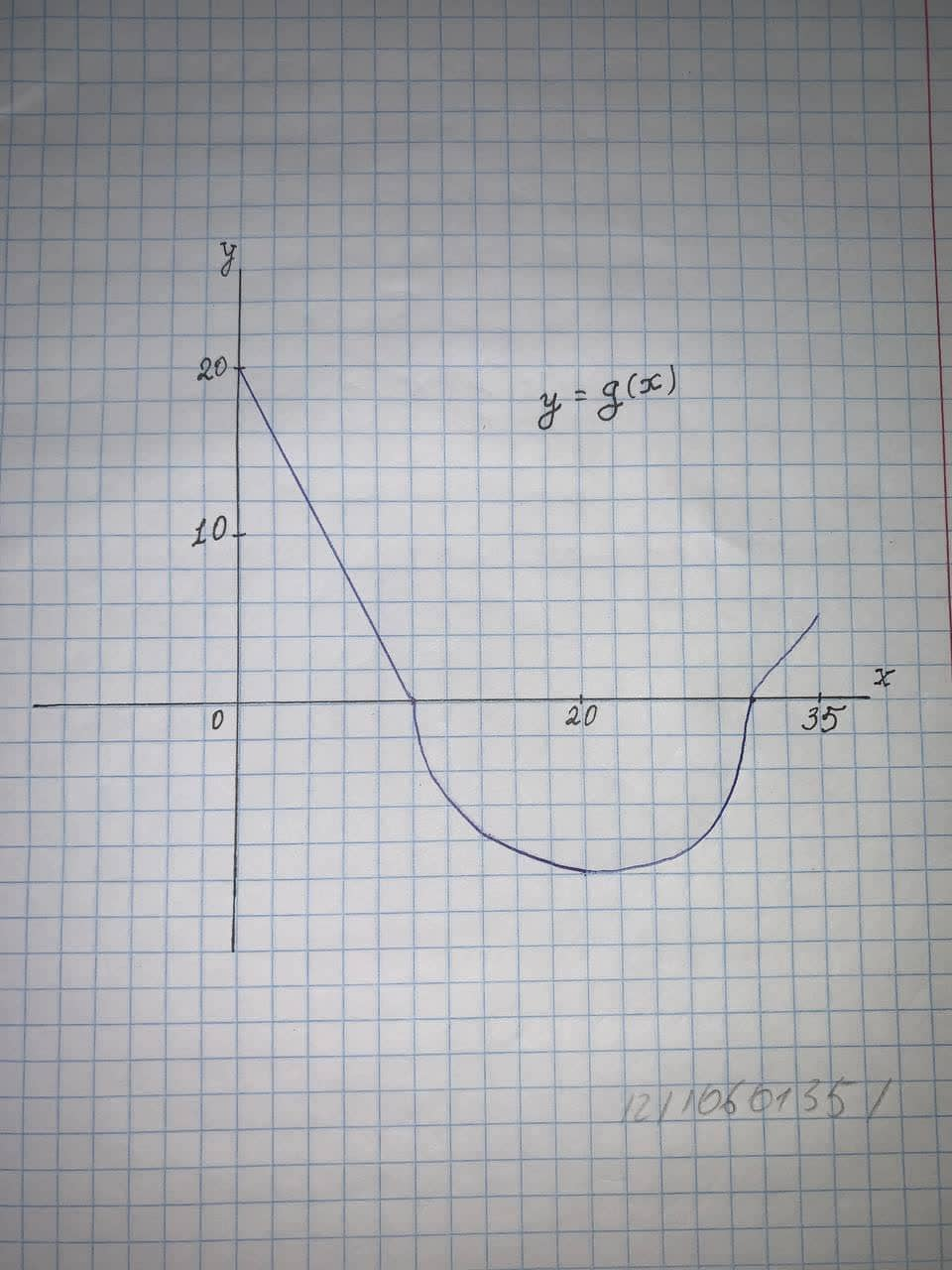# the graph of g consists of two straight lines and a semicircle. use it to evaluate each integral12110601351.jpga)\int_0^{10}g(x)dxb)\int_{10}^{30}g(x)dxc)\int_0^{35}g(x)dxarenceabigns 2021-05-17 Answered

the graph of g consists of two straight lines and a semicircle. use it to evaluate each integrala)${\int }_{0}^{10}g\left(x\right)dx$
b)${\int }_{10}^{30}g\left(x\right)dx$
c)${\int }_{0}^{35}g\left(x\right)dx$

You can still ask an expert for help

• Questions are typically answered in as fast as 30 minutes

Solve your problem for the price of one coffee

• Math expert for every subject
• Pay only if we can solve itDemi-Leigh Barrera

we have to evaluate the integral from the given graph
we know that integration of the function f(x) is nothing but the area under the curve f(x).
a)
we have to evaluate,
${\int }_{0}^{10}g\left(x\right)dx$
In the graph of g(x) we can see that between x = 0 and x = 10 g(x) is nothing but the right angled triangle with base 10 and height 20
we know that the area of right angled triangle is given by,

hence we can say that the area of right angled triangle with base 10 and height 20 is given by
$A=\frac{1}{2}\cdot 10\cdot 20=100$
Hence we can say that,

$⇒{\int }_{0}^{10}g\left(x\right)dx=100$
b)we have to evaluate,
${\int }_{10}^{30}g\left(x\right)dx$
In the graph of g(x) we can see that between x = 10 and x = 30 g(x) is nothing but the semicircle with radius 10
we know that area of a semicircle with radius r is given by,
$A=\frac{1}{2}\pi {r}^{2}$
Hence we can say that area of a semicircle with radius 10 is given by,
$A=\frac{1}{2}\pi \left(10{\right)}^{2}=\frac{1}{2}\pi \cdot 100=50\pi$
But we can see that semicircle is below x axis hence we can say that,

$⇒{\int }_{10}^{30}g\left(x\right)dx=-50\pi$
c)
we have to evaluate,
${\int }_{0}^{35}g\left(x\right)dx$

we can say that,

${\int }_{0}^{35}g\left(x\right)dx={\int }_{0}^{10}g\left(x\right)dx+{\int }_{10}^{30}g\left(x\right)dx+{\int }_{30}^{35}g\left(x\right)dx$

we have,

${\int }_{0}^{10}g\left(x\right)dx=100,{\int }_{10}^{30}g\left(x\right)dx=-50\pi$

Hence we can say that,

${\int }_{0}^{35}g\left(x\right)dx=100-50\pi +{\int }_{30}^{35}g\left(x\right)dx$

now we will evaluate,

${\int }_{30}^{35}g\left(x\right)dx$

In the graph of g(x) we can see that between x = 30 and x = 35 g(x) is nothing but the right angled triangle with base 5 and height 5
we know that the area of right angled triangle is given by,

hence we can say that the area of right angle triangle with base 5 and height 5 is given by,
$A=\frac{1}{2}\cdot 5\ast 5=\frac{25}{2}$
Hence we can say that,

$⇒{\int }_{30}^{35}g\left(x\right)dx=\frac{25}{2}$
Put this value in equation 1) we can say that,
${\int }_{0}^{35}g\left(x\right)dx=100-50\pi +\frac{25}{2}$
$⇒{\int }_{0}^{35}g\left(x\right)dx=\frac{200+25}{2}-50\pi$
$⇒{\int }_{0}^{35}g\left(x\right)dx=\frac{225}{2}-50\pi$